Parents and Teachers: Support Ducksters by following us onor.

Kids Math

Finding Surface Area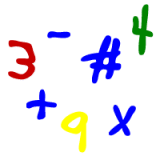Skills needed:
Multiplication
Subtraction
Division
Polygons

In this section we'll cover the surface area of two-dimensional objects like squares, rectangles, and triangles. The surface area is the total exposed area inside a given boundary. We write area in units squared.

Here is an example of surface area using a square:

This square is 4 units long on each side. The surface area is the number of square units that fit into the square. As shown in the picture, the surface area of this square is 16 total square units.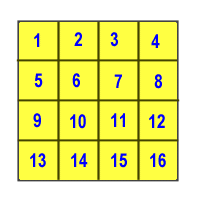With a rectangle and square we can also get the surface area by multiplying width (W) x length (L). Let's try that and see if we get the same answer:

Area = W x L
Area = 4 x 4
Area = 16

Note: if the units were feet for this problem, the answer would be 16 feet squared. Not just 16 feet. When we give the answer for surface area we used squared to indicate that it's a surface area and not just a straight line.

Let's take the more complicated example of this football field. We used this same example to demonstrate how to figure the perimeter (see perimeter for kids). The perimeter of this football field is the sum of all the sides 100 + 50 + 100 + 50 = 300 yards.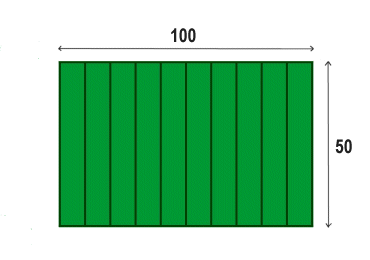What is the surface area using yards for the units? Since this is a rectangle we can use the rectangle formula:

Area = W x L
Area = 100 yards x 50 yards
Area = 5000 yards squared

Find the surface area of this polygon: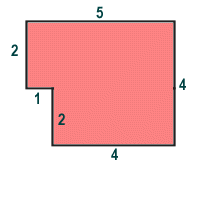This looks confusing at first, but we can make this easier by dividing it up into two rectangles like this: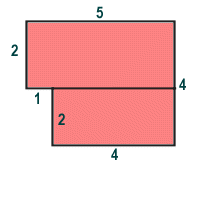Now we can add the surface area of the two rectangles:

The top rectangle is 2 x 5 = 10.
The bottom rectangle is 2 x 4 = 8
The total surface area is 10 + 8 = 18.

We could have also divided it up into these two different rectangles. Try this and see if you get the same answer.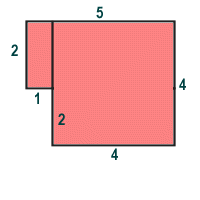4 x 4 = 16
2 x 1 = 2
16 + 2 = 18.

Figure the surface area of a triangle

To figure out the surface area of a triangle, we need to know the base and the height. The base is any side we choose. The height is the distance from the vertex opposite to the base at a 90 degree angle to the base. Okay, this is a little tricky, but it makes more sense looking at the picture below. The base is b and the height is h.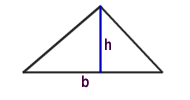Once we have the base and height, we can use the following formula:

Area of a triangle = ½ (b x h)

Example:

Find the surface area of this triangle: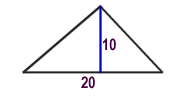Area = ½ (b x h)
Area = ½ (20 x 10)
Area = ½ (200)
Area = 100

In the case of a right triangle, the base and height are the two sides that are perpendicular or at 90 degrees to each other.

More Geometry Subjects

Circle
Polygons
Triangles
Pythagorean Theorem
Perimeter
Slope
Surface Area
Volume of a Box or Cube
Volume and Surface Area of a Sphere
Volume and Surface Area of a Cylinder
Volume and Surface Area of a Cone
Angles glossary
Figures and Shapes glossary

Back to Kids Math

Back to Kids StudyFollow us onor